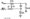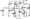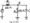# Electronic Circuits July 2008

Note : Answer any FIVE full questions, choosing atleast two questions from each part.

PART-A

1 a. Explain the working of a negative clipper with its ct diagram.

b. Sketch the wave form output Vout in the following circuit, indicating the values of maximum positive and negative output voltages.c.  Explain the working of a positive clamper with suitable diagrams.

d.  Explain the working of Schottkey diode.

2 a. What is the ac collector voltage in the first stage of the amplifier circuit shown in Fig. Q 2(a)? Calculate the output voltage Vout across the load resistor.b. Draw the circuit diagram of a swamped amplifier. Write down its ac equivalent circuit and derive expressions for Zin (base) and voltage gain Av.

3 a. With the help of a circuit diagram, explain the working of a voltage divider biased (VDB) amplifier, highlighting on bias stabilization.

b.  In the following circuit, what is the value of capacitor ‘C’ needed to efficiently short the point E to ground, if the input frequency is 1 kHz?c. Write down the circuit diagram of a base – biased amplifier and its ac equivalent circuit replacing the transistor by its % model.

4 a. Explain the working of an emitter follower circuit with its circuit diagram and its ac equivalent circuit.

b.  Distinguish between class A, class B, class AB and class C amplifier.

c.  Calculate the voltage gain and the ac voltage of the following emitter follower if p = 150.Fig. Q 4(c)

PART-B

5 a. Explain the structure of the depletion mode MOSFET. And the D – MOSFET curves.

b.  Explain the active load switching circuit using the MOSFET. Draw its equivalent circuit and its two terminal curves.

6 a. Explain the frequency response of an amplifier. Describe the effect of the components that reduces the response of the amplifier below and above the midland.

b.  Explain the four types of negative feedback amplifiers.

7 a. Draw the circuit diagram of an integrator and explain its operation with a typical input pulse.

b.  Draw and explain the circuit diagram of voltage controlled oscillator using the 555 timer.

8 a. Define the terms ‘Load Regulation’ and ‘Line Regulation’ with respect to a power supply.

b. Describe with circuit diagram, the operation of a shunt regulation power supply.

c.  What are the advantages and disadvantages of shunt regulator? What are the advantages of a series regulator?